# WBJEE Subjective Mathematics Sample Paper III Notes | Study WBJEE Sample Papers, Section Wise & Full Mock Tests - JEE

## JEE: WBJEE Subjective Mathematics Sample Paper III Notes | Study WBJEE Sample Papers, Section Wise & Full Mock Tests - JEE

The document WBJEE Subjective Mathematics Sample Paper III Notes | Study WBJEE Sample Papers, Section Wise & Full Mock Tests - JEE is a part of the JEE Course WBJEE Sample Papers, Section Wise & Full Mock Tests.
All you need of JEE at this link: JEE

Question: 1. A train moving with constant acceleration takes t seconds to pass a certain fixed point and the front and back end of the train pass the fixed point with velocities u and v respectively. Show that the length of the trai is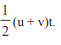Ans: v = u + at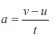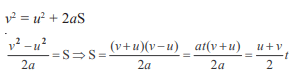Question: 2. Show that​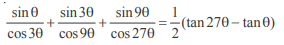Ans: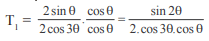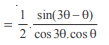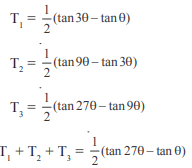Question: 3. If x = sin t, y = sin 2t, prove that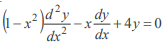Ans: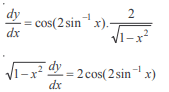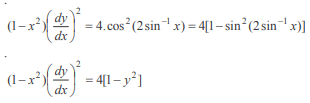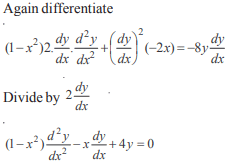Question: 4. Show that, for a positive integer n, the coefficient of xk (0 ≤ K ≥ n) in the expansion of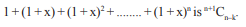Ans: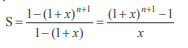Coefficient of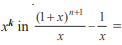Coefficient of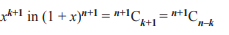Question: 5. If m, n be integers, then find the value of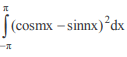Ans: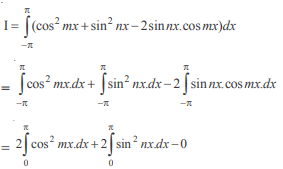(Odd .....)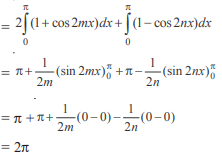Question: 6. Find the angle subtended by the double ordinate of length 2a of the parabola y2 = ax at its vertex
Ans: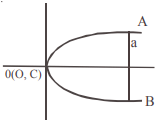y2 = ax, a2 = ax, a = x    [ put y = a]
A (a, a), B(a, –a)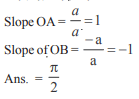Question: 7.  If f is differentiable at x = a, find the value of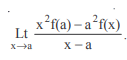Ans: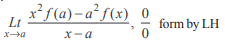Question: 8. Find the values of ‘a’ for which the expression x2 – (3a – 1)x + 2a2 + 2a – 11 is always positve.
Ans: x2 – (3a – 1) x + 2a2 + 2a – 11 > 0
D < 0
(3a – 1)2 – 4 (2a2 + 2a –11) < 0
9a2 – 6a + 1 – 8a2 – 8a + 44 < 0
a2 – 14a + 45 < 0
(a – 9) (a – 5) < 0
5 < a < 9

Question: 9. Find the sum of the first n terms of the series 0.2 + 0.22 + 0.222 + ..........
Ans: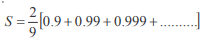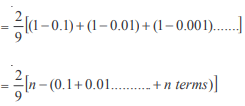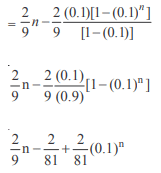Question: 10. The equation to the pairs of opposite sides of a parallelogram are x2 – 5x + 6 = 0 and y2 – 6y + 5. Find the equations of its diagonals.
Ans: x = 2 ......(i)
x = 3 ...... (ii)
y = 1 .... (iii)
y = 5 ..... (iv)
A (2, 1), B (3, 1), C (3, 5), D(2, 5)
Equation of AC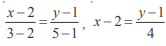4x – 8 = y – 1, 4x – y – 7 = 0
Equation of BD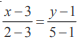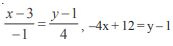4x + y – 13 = 0

The document WBJEE Subjective Mathematics Sample Paper III Notes | Study WBJEE Sample Papers, Section Wise & Full Mock Tests - JEE is a part of the JEE Course WBJEE Sample Papers, Section Wise & Full Mock Tests.
All you need of JEE at this link: JEEUse Code STAYHOME200 and get INR 200 additional OFF

## WBJEE Sample Papers, Section Wise & Full Mock Tests

4 videos|10 docs|54 tests

Track your progress, build streaks, highlight & save important lessons and more!

,

,

,

,

,

,

,

,

,

,

,

,

,

,

,

,

,

,

,

,

,

,

,

,

;